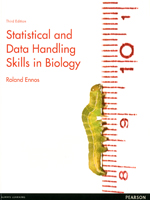Statistical & Data Handling Skills in Biology, 3rd edition

By Roland Ennos
April 2012
Pearson Education
ISBN: 9780273729495
Distributed by Trans-Atlantic Publications
271 pages, Illustrated
\$59.50 Paper OriginalThe ability to expertly analyse statistical data is a crucial skill in the biological sciences – it is fundamental to fully understanding what your experiments are actually telling you.

Statistical and Data Handling Skills in Biology gives you everything you need to master statistical analysis. Written in a straight-forward and easy to understand style it presents all of the tests you will need throughout your studies, and shows you how to choose the right tests to get the most out of your experiments. All of this is done in the context of biological examples so you can see just how relevant a skill this is, and how mastering it will make you a more rounded scientist.

Contents:

An Introduction to Statistics
1.1 Becoming a research biologist
1.2 Awkward questions
1.3 Why do biologists have to repeat everything?
1.4 Why do biologists have to bother with statistics?
1.5 Why is statistical logic so strange?
1.6 Why are there are so many statistical tests?
1.7 Using the decision chart
1.8 Using this book

2 Dealing with variation
2.1 Introduction
2.2 Examining the distribution of data
2.3 The normal distribution
2.4 Describing the normal distribution
2.5 The variability of samples
2.6 Confidence limits
2.7 Presenting descriptive statistics and confidence limits
2.8 Introducing computer packages
2.9 Calculating descriptive statistics
2.10 Self assessment problems

3 Testing for normality and transforming data
3.1 The importance of normality testing
3.2 The Kolgomorov-Smirnov test
3.3 What to do if your data has a significantly different distribution from the normal
3.4 Examining data in practice
3.5 Transforming data
3.6 The complete testing procedure
3.7 Self-assessment problems

4 Testing for differences between one or two groups
4.1 Introduction
4.2 Why we need statistical tests for differences
4.3 How we test for differences
4.4 One and two tailed tests
4.5 The types of test and their non parametric equivalents
4.6 The one sample t test
4.7 The paired t test
4.8 The two-samplet test
4.9 Introduction to non parametric tests for differences
4.10 The one sample sign test
4.11 The Wilcoxon matched pairs test
4.12 The Mann-Whitney U test
4.13 Self assessment problems

5 Testing for difference between more than two groups: ANOVA and its non parametric equivalents
5.1 Introduction
5.2 One way ANOVA
5.3 Deciding which groups are different – post hoc tests
5.4 Presenting the results of one way ANOVA’s
5.5 Repeated measures ANOVA
5.6 The Kruskall-Wallis test
5.7 The Friedman test
5.8 Two way ANOVA
5.9 The Scheirer-Ray-Hare test
5.10 Nested ANOVA
5.11 Self assessment problems

6 Investigating relationships
6.1 Introduction
6.2 Examining data for relationships
6.3 Examining graphs
6.4 Linear relationships
6.5 Statistical tests for linear relationships
6.6 Correlation
6.7 Regression
6.8 Studying common non-linear relationships
6.9 Dealing with non normally distributed data: rank correlation
6.10 Self assessment problems

7 Dealing with Categorical Data
7.1 Introduction
7.2 The problem of variation
7.3 The ÷2 test for differences
7.4 The ÷2 test for associations
7.5 Validity of ÷2 tests
7.6 Logistic regression
7.7 Self assessment problems

8 Designing Experiments
8.1 Introduction
8.2 Preparation
8.3 Excluding confounding variables
8.4 Replication and pseudoreplication
8.5 Randomisation and blocking
8.6 Choosing the statistical test
8.7 Choosing the number of replicates: power calculations
8.9 Self assessment problems

9 More complex statistical analysis
9.1 Introduction to complex statistics
9.2 Experiments investigating several factors
9.3 Experiments in which you cannot control all the variables
9.4 Investigating the relationships between several variables
9.5 Exploring data to investigate groupings

10 Dealing with measurements and units
10.1 Introduction
10.2 Measuring
10.3 Converting to SI units
10.4 Combining values
10.6 Doing all three steps
10.7 Constants and formulae
10.8 Using calculations
10.9 Logarithms, graphs and pH
10.10 Self assessment problems

Glossary

Solutions

Statistical Tables
Table S1: Critical values for the statistic
Table S2: Critical values for the correlation coefficient r
Table S3: Critical values for the c2statistic
Table S4: Critical values for the Wilcoxon T statistic
Table S5: Critical values for the Mann-Whitney U statistic
Table S6: Critical values for the Friedman c2statistic
Table S7: Critical values for the Spearman rank correlation coefficient r